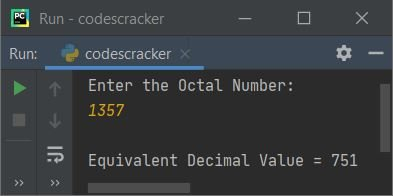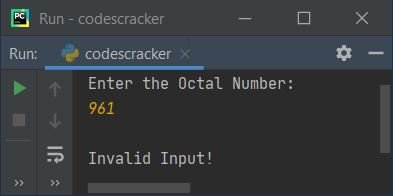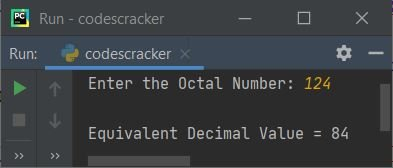# Python Program to Convert Octal to Decimal

This article is created to cover some programs in Python, to convert octal number entered by user to its equivalent decimal value. Here are the list of programs:

• Octal to Decimal using while Loop
• Using int() Method
• Using user-defined Function
• Using Class and Object

Note - Before creating these programs, if you're not aware about steps used for the conversion, then refer to Octal to Decimal Steps, Formula, Example to get every required things about the topic.

## Octal to Decimal using while Loop

To convert octal to decimal number in Python, you have to ask from user to enter a number in octal number system, then convert that number into its equivalent decimal value as shown in the program given below:

The question is, write a Python program to convert octal to decimal using while loop. Here is its answer:

```print("Enter the Octal Number: ")
octnum = int(input())

chk = 0
i = 0
decnum = 0
while octnum!=0:
rem = octnum%10
if rem>7:
chk = 1
break
decnum = decnum + (rem * (8 ** i))
i = i+1
octnum = int(octnum/10)

if chk == 0:
print("\nEquivalent Decimal Value =", decnum)
else:
print("\nInvalid Input!")```

Here is the initial output produced by this Python program:Now supply the input say 1357 as octal number, and press `ENTER` key to convert and print its equivalent decimal value as shown in the snapshot given below:Here is another sample run with octal number input 961:Note - 9 is an invalid octal digit.

The dry run of above program with user input 1357 goes like:

• Initial values, octnum=1357 (entered by user), chk=0, i=0, decnum=0
• Now the condition (of while loop) octnum!=0 or 1357!=0 evaluates to be true, therefore program flow goes inside the loop
• octnum%10 or 1357%10 or 7 gets initialized to rem
• Now the condition (of if) rem>7 or 7>7 evaluates to be false, therefore program flow does not goes inside this if's body. This condition is created to check for invalid octal digit. Since 8 and 9 are both invalid octal digits, therefore if any digit found to be 8 or 9, then using break keyword, the execution of while loop gets ended and 1 gets initialized to chk
• Since the condition of if (inner) evaluates to be false, therefore program flow does not goes in its body. Rather decnum + (rem * (8 ** i)) or 0 + (7 * (8 ** 0)) or 7 * 80 or 7*1 or 7 gets initialized to decnum
• i+1 or 0+1 or 1 gets initialized to i
• int(octnum/10) or int(1357/10) or 135 gets initialized to octnum
• Now program flow goes back and evaluates the condition of while loop again with new value of octnum.
• That is, the condition octnum!=0 or 135!=0 evaluates to be true again, therefore program flow again goes inside the loop. This process continues until the condition evaluates to be false
• In this way, the given octal value gets converted into its equivalent decimal value
• Therefore after exiting from the loop, print the value of decnum as decimal equivalent
• Before printing the value, I've applied the condition to check whether the value of chk is 0 (its original or initial value) or not
• If it equals 0, means that, program flow never goes inside the if's body in while loop. That means, all digits of given number are valid octal digits

## Octal to Decimal using int()

This program uses int() method to convert octal number to decimal. The int() method returns integer (decimal) equivalent of value passed as its argument. The end= used in the program given below, to skip printing of an automatic newline using print():

```print("Enter the Octal Number: ", end="")
onum = input()

dnum = int(onum, 8)
print("\nEquivalent Decimal Value =", dnum)```

Here is its sample run with user input 124:## Octal to Decimal using Function

This program is created using user-defined function named OctToDec(). This function takes a value (octal) as its argument and returns its decimal equivalent using int() method.

```def OctToDec(o):
return int(o, 8)

print("Enter the Octal Number: ", end="")
onum = input()

dnum = OctToDec(onum)
print("\nEquivalent Decimal Value =", dnum)```

## Octal to Decimal using Class

This program is created using a class, an object-oriented feature of Python. In this program, an object obj is created of class CodesCracker to access its member function OctToDec() using dot (.) operator. The member function OctToDec() returns the decimal equivalent of octal value passed as its argument.

```class CodesCracker:
def OctToDec(self, o):
return int(o, 8)

print("Enter the Octal Number: ", end="")
onum = input()

obj = CodesCracker()
dnum = obj.OctToDec(onum)
print("\nEquivalent Decimal Value =", dnum)```

#### Same Program in Other Languages

Python Online Test

« Previous Program Next Program »